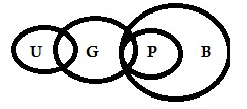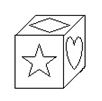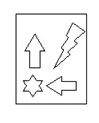# SSC CGL 2018 Practice Test Papers | Reasoning Ability (Day-5)

Dear Aspirants, Here we have given the Important SSC CGL Exam 2018 Practice Test Papers. Candidates those who are preparing for SSC CGL 2018 can practice these questions to get more confidence to Crack SSC CGL 2018 Examination.

[WpProQuiz 2673]

Click “Start Quiz” to attend these Questions and view Explanation

1. Four years ago, the age of Sonu was equal to the present age of Mahi. Sum of Sonu’s age 5 year ago and Mahi’s age 6 years later is 81 years. If Ruchi is 4 years elder to Sonu, what will be Ruchi’s age (in years) after 10 years?
1. 54
2. 46
3. 48
4. 56

2). In the following question, from the given alternative words, select the word which can be formed using the letters of the given word.

NOTORIOUS

1. TORTOISE
2. SERIOUS
3. SOUR
4. STRING
1. In a certain code language, “BREWERY” is written as “ZSFXFSC” and “EPICURE” is written as “FSVDJQF”. How is “STEVEDORE” written in that code language?
1. FSPEFFWUT
2. FSPEFWFUT
3. FSPWFEFUT
4. TSPEFWFUF
1. If “α” denotes “subtraction”, “β” denotes “multiplication”, “θ” denotes “addition” and “δ” denotes “division”, then

20 θ 12 β 4 δ 24 α 9 = ?

1. 7
2. 13
3. 5
4. 11
1. If 13*9+12=117108 and 7*13+11=91143, then 14*8+13=?
1. 112104
2. 114102
3. 104112
4. 401112
1. In the following question. select the number which can be placed at the sign of question mark (?) from the given alternatives.
 8 10 6 3 5 4 12 ? 16
1. 15
2. 25
3. 20
4. 18

7). How many quadrilaterals are there in the given figure?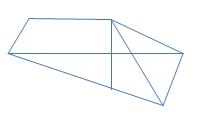1. 8
2. 4
3. 5
4. 6

8). In each of the following question below are given some statements followed by some conclusions. Taking the given statements to be true even if they seem to be at variance from commonly known facts, read all the conclusions and then decide which of the given conclusion logically follows the given statements.

Statements:

1. Some Girls are pretty.
2. All Pretty is Beautiful.
3. Some ugly are Girls.

Conclusions:

1. Some girls are not beautiful.
2. Some girls are ugly.
3. Some Beautiful is girls.
1. Only conclusion (I) and (II) follow.
2. Only conclusion (II) follows.
3. Only conclusion (II) and (III) follow.
4. All conclusions follow.
1. From the given options which answer figure can be formed by folding the figure given in the question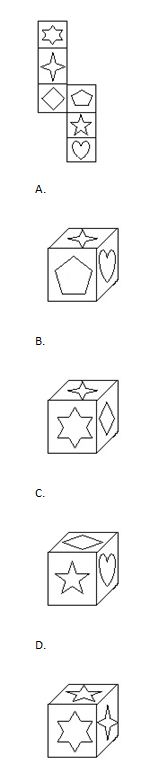1. If a mirror is placed on the line AB, then which of the answer figures is the right image of the given figure?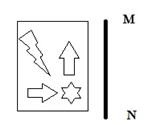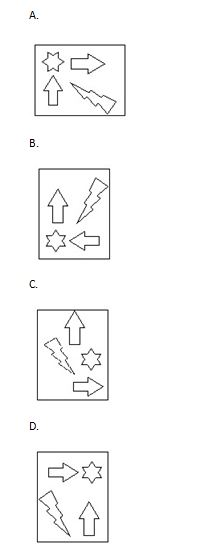S-4=M                     => S-M=4 …… (1)

S-5+M+6=81         => S+M=80…… (2)

By solving (1) & (2) We get Sonu and Mahi’s present age ie., Sonu =42, Mahi is 38.

Ruchi = Mahi +4 = 42+4=46.

10 years later 46+10=56.

Other than the option C can’t be formed using the word using NOTORIOUS.

coding follows the given Logic ,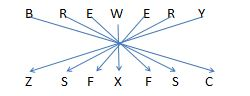By solving the sum according to the BODMAS Rule, 20+12*4/24-9=13

13*9=117, 12*9=108 => 117108

7*13=91, 11*13=143 =>91143

Similarly, 14*8=112, 13*8=104 =>112104

82+62=102

32+42=52

122+162=202

There are eight quadrilaterals in the given pic.

Only conclusion (II) and (III) follow